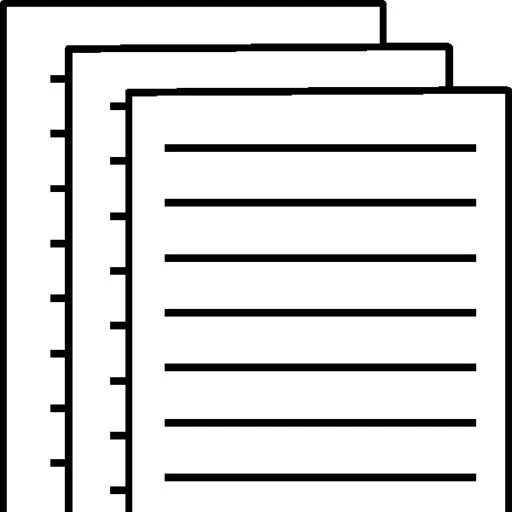## Array Manipulation

Array Manipulation

Numbers Reversed

PRG 211

University of Phoenix

Array Manipulation

This program will start by having the user input 10 random numbers. After the 10th number is inputted the program will output the average of the numbers inputted. After this the program will display the numbers the user inputted earlier from largest to smallest in descending order.

Pseudocode

Begin

DeclareMyArrayNumbers 

Set total = 0

For Counter1 to 10

Input Numbers(Counter)

Set total = total + Numbers(Counter)

End For

For Order1 to 10-1

For N 1 to 10-1

If Numbers(N)> Numbers(N + 1)

Set Temp = Numbers(N)

Set Numbers(N) = Numbers(N + 1)

Set Numbers(N + 1) = Temp

End For

Set average = total/10

Write “Average total of all numbers are:” + average

Write “The numbers reversed are:”

For N 10 to 1 by -1

Write Numbers(N)

End for

End

To view and download a complete answer, scroll down to the bottom to pay